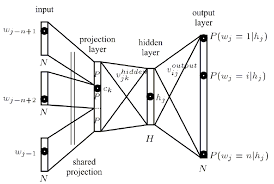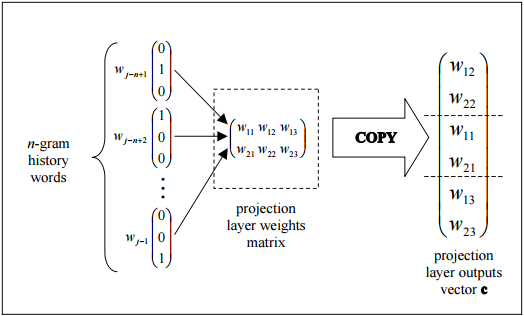2 views

I am currently trying to understand the architecture behind the word2vec neural net learning algorithm, for representing words as vectors based on their context.

After reading Tomas Mikolov paper I came across what he defines as a projection layer. Even though this term is widely used when referred to word2vec, I couldn't find a precise definition of what it actually is in the neural net context.My question is, in the neural net context, what is a projection layer? Is it the name given to a hidden layer whose links to previous nodes share the same weights? Do its units actually have an activation function of some kind?

￼Another resource that also refers more broadly to the problem can be found in this tutorial, which also refers to a projection layer around page 67.

by (33.1k points)

To be more clear, in natural language processing, traversing over two words sequentially in a text paragraph is called a bi-gram problem. The projection layer maps the discrete word indices of an n-gram context to a continuous vector space.

The projection layer is shared such that for contexts containing the same word multiple times, the same set of weights is applied to form each part of the projection vector. This organization effectively increases the amount of data available for training the projection layer weights since each word of each context training pattern individually contributes changes to the weight values.Each neuron in the projection layer is represented by a number of weights equal to the size of the vocabulary. The projection layer differs from the hidden and output layers by not using a non-linear activation function. Its purpose is simply to provide an efficient means of projecting the given n-gram context onto a reduced continuous vector space for subsequent processing by hidden and output layers trained to classify such vectors.